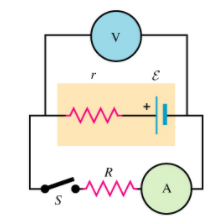# Problem: Measuring the EMF and Internal Resistance of a Battery When switch S in the figure is open, the voltmeter V of the battery reads 3.09 V . When the switch is closed, the voltmeter reading drops to 2.97 V , and the ammeter A reads 1.69 A . Assume that the two meters are ideal, so they do not affect the circuita) Find the emf E.Express your answer in volts to three significant digits.b) Find the internal resistance of the batteryc) Find the circuit resistance R.

###### FREE Expert Solution

This problem involves multiple concepts learned in circuits and resistance.

The equation for the terminal voltage is:

$\overline{){\mathbf{V}}{\mathbf{=}}{\mathbf{\epsilon }}{\mathbf{-}}{\mathbf{i}}{\mathbf{r}}}$ where V is the terminal voltage, ε is the emf and r is the internal resistance.

Ohm's law:

$\overline{){\mathbf{V}}{\mathbf{=}}{\mathbf{i}}{\mathbf{R}}}$

81% (397 ratings)###### Problem Details

Measuring the EMF and Internal Resistance of a Battery

When switch S in the figure is open, the voltmeter V of the battery reads 3.09 V . When the switch is closed, the voltmeter reading drops to 2.97 V , and the ammeter A reads 1.69 A . Assume that the two meters are ideal, so they do not affect the circuita) Find the emf E.
Express your answer in volts to three significant digits.

b) Find the internal resistance of the battery

c) Find the circuit resistance R.

Frequently Asked Questions

What scientific concept do you need to know in order to solve this problem?

Our tutors have indicated that to solve this problem you will need to apply the Solving Resistor Circuits concept. You can view video lessons to learn Solving Resistor Circuits. Or if you need more Solving Resistor Circuits practice, you can also practice Solving Resistor Circuits practice problems.